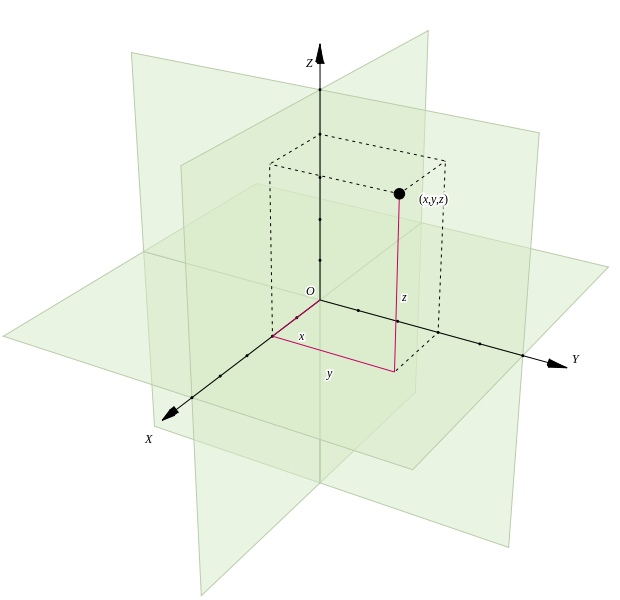# Worksheet 00: Vectors and Coordinate Systems

$$\newcommand{\vecs}{\overset { \rightharpoonup} {\mathbf{#1}} }$$ $$\newcommand{\vecd}{\overset{-\!-\!\rightharpoonup}{\vphantom{a}\smash {#1}}}$$$$\newcommand{\id}{\mathrm{id}}$$ $$\newcommand{\Span}{\mathrm{span}}$$ $$\newcommand{\kernel}{\mathrm{null}\,}$$ $$\newcommand{\range}{\mathrm{range}\,}$$ $$\newcommand{\RealPart}{\mathrm{Re}}$$ $$\newcommand{\ImaginaryPart}{\mathrm{Im}}$$ $$\newcommand{\Argument}{\mathrm{Arg}}$$ $$\newcommand{\norm}{\| #1 \|}$$ $$\newcommand{\inner}{\langle #1, #2 \rangle}$$ $$\newcommand{\Span}{\mathrm{span}}$$ $$\newcommand{\id}{\mathrm{id}}$$ $$\newcommand{\Span}{\mathrm{span}}$$ $$\newcommand{\kernel}{\mathrm{null}\,}$$ $$\newcommand{\range}{\mathrm{range}\,}$$ $$\newcommand{\RealPart}{\mathrm{Re}}$$ $$\newcommand{\ImaginaryPart}{\mathrm{Im}}$$ $$\newcommand{\Argument}{\mathrm{Arg}}$$ $$\newcommand{\norm}{\| #1 \|}$$ $$\newcommand{\inner}{\langle #1, #2 \rangle}$$ $$\newcommand{\Span}{\mathrm{span}}$$$$\newcommand{\AA}{\unicode[.8,0]{x212B}}$$

Name: ______________________________

Section: _____________________________

Student ID#:__________________________

Vectors have both magnitude and direction. We often describe them by separating into their component parts, in terms of unit vectors along the x, y, and z directions, i.e., $$\hat{i}$$, $$\hat{j}$$, and $$\hat{k}$$, respectively, as shown below## Q1: Vectors in Cartesian Coordinates

How do you write an arbitrary vector $$\vec{A}$$ in terms of its $$x$$, $$y$$, and $$z$$ components?

How do you calculate the length of vector $$\vec{A}$$: length=$$|\vec{A}|$$?

On the axes to the left below, draw the vector $$\vec{B}=2\hat{i}-\hat{j}+3\hat{k}$$. Then draw $$\vec{C}=-\hat{i}+2\hat{j}-\hat{k}$$. You will want to include some tick marks to help you to draw.

What is $$\vec{B}-\vec{C}$$? Show this vector on the axes to the right. How long is this vector?

## Q2: Vectors in Spherical Coordinants

Cartesian coordinates, $$x,y,z$$, are the common coordinates we frequently use but sometimes they are not the best ones to choose. In many problems, spherical polar coordinates are better. Use the figure below to describe the length of vector $$\vec{r}$$ in terms of $$x$$,$$y$$, and $$z$$?

What is $$x$$ in terms of $$r, \theta$$, and $$\phi$$?

What is $$y$$ in terms of $$r, \theta$$, and $$\phi$$?

What is $$z$$ in terms of $$r, \theta$$, and $$\phi$$?

## Q3: Volume Elements

What is the expression for the volume element used in 3D integrals: $$dV=dx\,dy\,dz$$?

Does the figure below confirm the volume element you determine above?

Does your volume element yield the correct value for the volume of a sphere if you perform the integral, $$V=\int dV$$? What is the explicit form of this integral in terms of $$r, \theta$$ and $$\phi$$?
Why is $$dr\,d\theta\, d\phi$$ alone not the appropriate volume element?
What is expression used to evaluate the average value of $$\cos{\theta}$$ over the surface of a sphere?
What is expression used to evaluate the average of $$\cos^2{\theta}$$ over the surface of a sphere?